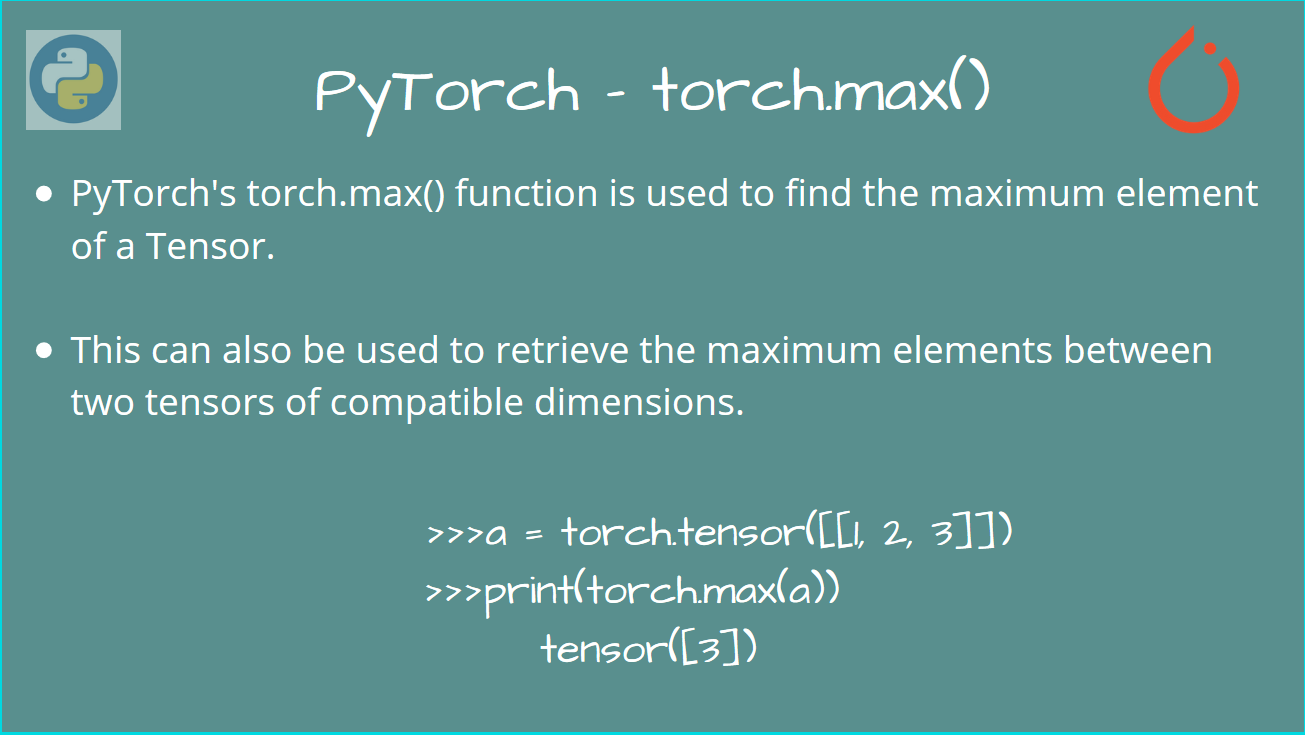# How to use the PyTorch torch.max()

Published on August 3, 2022By Vijaykrishna RamWhile we believe that this content benefits our community, we have not yet thoroughly reviewed it. If you have any suggestions for improvements, please let us know by clicking the “report an issue“ button at the bottom of the tutorial.

In this article, we’ll take a look at using the PyTorch torch.max() function.

As you may expect, this is a very simple function, but interestingly, it has more than you imagine.

Let’s take a look at using this function, using some simple examples.

NOTE: At the time of writing, the PyTorch version used is PyTorch 1.5.0

## PyTorch torch.max() - Basic Syntax

To use PyTorch `torch.max()`, first import `torch`.

``````import torch
``````

Now, this function returns the maximum among the elements in the Tensor.

### Default Behavior of PyTorch torch.max()

The default behavior is to return a single element and an index, corresponding to the global maximum element.

``````max_element = torch.max(input_tensor)
``````

Here is an example:

``````p = torch.randn([2, 3])
print(p)
max_element = torch.max(p)
print(max_element)
``````

Output

``````tensor([[-0.0665,  2.7976,  0.9753],
[ 0.0688, -1.0376,  1.4443]])
tensor(2.7976)
``````

Indeed, this gives us the global maximum element in the Tensor!

### Use torch.max() along a dimension

However, you may wish to get the maximum along a particular dimension, as a Tensor, instead of a single element.

To specify the dimension (axis - in `numpy`), there is another optional keyword argument, called `dim`

This represents the direction that we take for the maximum.

This returns a tuple, `max_elements` and `max_indices`.

• `max_elements` -> All the maximum elements of the Tensor.

• `max_indices` -> Indices corresponding to the maximum elements.

``````max_elements, max_indices = torch.max(input_tensor, dim)
``````

This will return a Tensor, which has the maximum elements along the dimension `dim`.

Let’s now look at some examples.

``````p = torch.randn([2, 3])
print(p)

# Get the maximum along dim = 0 (axis = 0)
max_elements, max_idxs = torch.max(p, dim=0)
print(max_elements)
print(max_idxs)
``````

Output

``````tensor([[-0.0665,  2.7976,  0.9753],
[ 0.0688, -1.0376,  1.4443]])
tensor([0.0688, 2.7976, 1.4443])
tensor([1, 0, 1])
``````

As you can see, we find the maximum along the dimension 0 (maximum along columns).

Also, we get the indices corresponding to the elements. For example,`0.0688` has the index `1` along column 0

Similarly, if you want to find the maximum along the rows, use `dim=1`.

``````# Get the maximum along dim = 1 (axis = 1)
max_elements, max_idxs = torch.max(p, dim=1)
print(max_elements)
print(max_idxs)
``````

Output

``````tensor([2.7976, 1.4443])
tensor([1, 2])
``````

Indeed, we get the maximum elements along the row, and the corresponding index (along the row).

## Using torch.max() for comparison

We can also use `torch.max()` to get the maximum values between two Tensors.

``````output_tensor = torch.max(a, b)
``````

Here, `a` and `b` must have the same dimensions, or must be “broadcastable” Tensors.

Here is a simple example to compare two Tensors having the same dimensions.

``````p = torch.randn([2, 3])
q = torch.randn([2, 3])

print("p =", p)
print("q =",q)

# Compare elements of p and q and get the maximum
max_elements = torch.max(p, q)

print(max_elements)
``````

Output

``````p = tensor([[-0.0665,  2.7976,  0.9753],
[ 0.0688, -1.0376,  1.4443]])
q = tensor([[-0.0678,  0.2042,  0.8254],
[-0.1530,  0.0581, -0.3694]])
tensor([[-0.0665,  2.7976,  0.9753],
[ 0.0688,  0.0581,  1.4443]])
``````

Indeed, we get the output tensor having maximum elements between `p` and `q`.

## Conclusion

In this article, we learned about using the torch.max() function, to find out the maximum element of a Tensor.

We also used this function to compare two tensors and get the maximum among them.

For similar articles, do go through our content on our PyTorch tutorials! Stay tuned for more!

Click below to sign up and get \$200 of credit to try our products over 60 days!

### Popular Topics

##### Join the Tech Talk##### Hollie's Hub for Good

Working on improving health and education, reducing inequality, and spurring economic growth? We’d like to help.